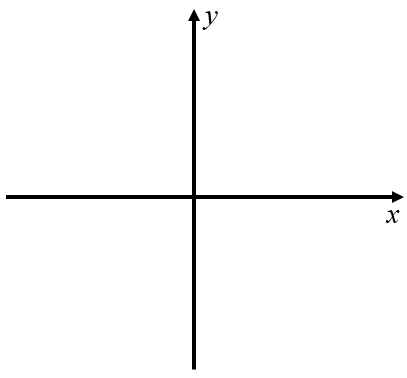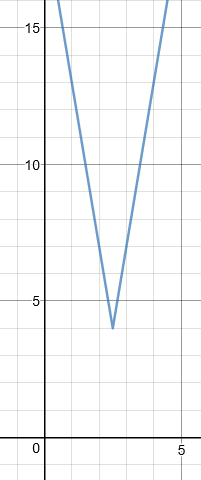# Exam-Style Questions.

## Problems adapted from questions set for previous Mathematics exams.

### 1.

GCSE Higher

At depths below 900 metres, the temperature of the water in the sea is given by the formula:

$$T=\frac{4500}{x}$$

Where $$x$$ is the depth in metres and the temperature of the water is T °C.

(a) A fish swims downwards from a depth of 1000 metres to a depth of 1500 metres. How many degrees colder is the sea water at 1500m than 1000m?(b) Roughly sketch the shape of a graph that shows that T is inversely proportional to $$x$$.

### 2.

GCSE Higher

The images below show a graphic display calculator screen with different functions displayed as graphs.

a) Which function is trigonometric?

b) Which function is inversely proportional to $$x$$?

c) Which function is exponential?

d) Which function is proportional to $$x^3$$?### 3.

GCSE Higher

Match the equation with the letter of its graphEquationGraph
$$y=3-\frac{10}{x}$$
$$y=2^x$$
$$y=\sin x$$
$$y=x^2+7x$$
$$y=x^2-8$$
$$y=2-x$$

### 4.

GCSE Higher

The following table shows corresponding values for two variables $$x$$ and $$y$$.

 x 1 2 3 4 y 5 $$1 \frac14$$ $$\frac{5}{9}$$ $$\frac{5}{16}$$

(a) If $$y$$ is inversely proportional to the square of $$x$$ find an equation for $$y$$ in terms of $$x$$.

(b) Find the positive value for $$x$$ when $$y = 20$$.

### 5.

GCSE Higher

(a) Sketch a graph on the axes below left that shows that $$y$$ is directly proportional to $$x$$.(b) Sketch the graph of $$y = -x^3$$ on the axes above right.

(c) It is possible to draw many rectangles that have area $$48 cm^2$$.

Draw a graph to show the relationship between length and width for rectangles with area $$48 cm^2$$ and sides less than $$50cm$$.

### 6.

GCSE Higher

At a constant temperature, the volume of a gas $$V$$ is inversely proportional to its pressure $$p$$. By what percentage will the pressure of a gas change if its volume increases by 15% ?

### 7.

IB Analysis and Approaches

The function $$f$$ is defined by:

$$f(x) = \frac{4x+2}{x+1}, \quad \text{ where } x \in \mathbb{R}, x \neq -1$$

(a) Write down the equation of the vertical asymptote of the graph of $$f$$.

(b) Write down the equation of the horizontal asymptote of the graph of $$f$$.

(c) Find the coordinates of the $$x$$-axis and $$y$$-axis intercepts.

(d) Sketch the graph of $$f$$.

### 8.

IB Analysis and Approaches

A function $$f$$ is defined by $$f(x) = 2 + \dfrac{1}{3-x}, \text{ where } x \in \mathbb{R}, x \neq 3.$$

The graph of $$y=f(x)$$ has a vertical asymptote and a horizontal asymptote.

(a) Write down the equation of the horizontal asymptote;

(b) Write down the equation of the vertical asymptote;

Find the coordinates of the point where the graph of $$y$$ intersects:

(c) the y-axis;

(d) the x-axis.

### 9.

IB Standard

Let $$f(x)=5x^2-20x+k$$. The equation $$f(x)=0$$ has two equal roots.

(a) Write down the value of the discriminant.

(b) Hence, show that $$k=20$$.

The graph of $$f$$ has its vertex on the x-axis.

(c) Write down the solution of $$f(x)=0$$.

(d) Find the coordinates of the vertex of the graph of $$f$$.

The function can be written in the form $$f(x)=a(x-h)^2+j$$.

(e) Find the value of $$a$$.

(f) Find the value of $$h$$.

(g) Find the value of $$j$$.

(h) The graph of a function $$g$$ is obtained from the graph of $$f$$ by a reflection in the x-axis, followed by a translation by the vector $$\begin{pmatrix} 0 \\ 3 \\ \end{pmatrix}$$. Find $$g$$, giving your answer in the form $$g(x)=Ax^2+Bx+C$$.

### 10.

A-LevelThe diagram on the right shows a sketch of part of the graph:

$$y = f(x) \quad \text{where} \quad f(x) = 3 | 5-2x | + 4$$

(a) State the range of $$f$$.

(b) Solve the equation $$f(x) = \frac{x}{3} + 20$$.

(c) Given that the equation $$f(x) = k$$, where $$k$$ is a constant, has two distinct roots, state the set of possible values for k.

### 11.

IB Standard

Let $$f(x) = \frac{9x-3}{bx+9}$$ for $$x \neq -\frac9b, b \neq 0$$.

(a) The line $$x = 3$$ is a vertical asymptote to the graph of $$f$$. Find the value of b.

(b) Write down the equation of the horizontal asymptote to the graph of $$f$$.

(c) The line $$y = c$$ , where $$c\in \mathbb R$$ intersects the graph of $$\begin{vmatrix}f(x) \end{vmatrix}$$ at exactly one point. Find the possible values of $$c$$.

If you would like space on the right of the question to write out the solution try this Thinning Feature. It will collapse the text into the left half of your screen but large diagrams will remain unchanged.

The exam-style questions appearing on this site are based on those set in previous examinations (or sample assessment papers for future examinations) by the major examination boards. The wording, diagrams and figures used in these questions have been changed from the originals so that students can have fresh, relevant problem solving practice even if they have previously worked through the related exam paper.

The solutions to the questions on this website are only available to those who have a Transum Subscription.

Exam-Style Questions Main Page

Search for exam-style questions containing a particular word or phrase:

To search the entire Transum website use the search box in the grey area below.